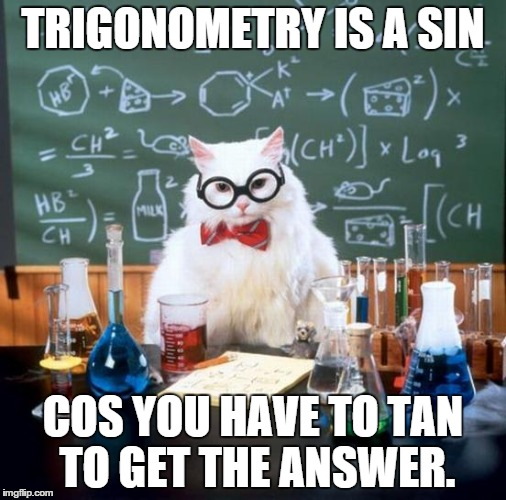# Mates y TIC - Maths and ICT

## Actividades de Matemáticas con TIC - Math Activities with ICT - - - (matesytic@gmail.com) Ricardo García Mesa

•## 3-gonometry-2

Posted by ricardogm on January 10th, 2019Hi

We have some things to do:

0. Solve these problems in your notebook while I try to correct your homework. Make drawings, of course.

1 The known data for a right triangle ABC is a = 5 m and B = 41.7°. Solve the triangle.

2 The known data for a right triangle ABC is b = 3 m and B = 54.6°. Solve the triangle.

3 The known data for a right triangle ABC is a = 6 m and b = 4 m. Solve the triangle.

4 The known data for a right triangle ABC is b = 3 m and c = 5 m. Solve the triangle.

5 A tree 50 m tall casts a shadow 60 m long. Find the angle of elevation of the sun at that time.

6 An airship is flying at an altitude of 800 m when it spots a village in the distance with a depression angle of 12°. How far is the village from where the plane is flying over?

7 Find the radius of a circle knowing that a chord of 24.6 m has a corresponding arc of 70°.

8 Calculate the area of a triangular field, knowing that two of its sides measure 80 m and 130 m and between them is an angle of 70°.

9 Calculate the height of a tree, knowing that from a point on the ground the top of the tree can be seen at an angle of 30º and from 10 m closer the top can be seen at an angle of 60°.

10 The length of the side of a regular octagon is 12 m. Find the radii of the inscribed and circumscribed circles.

11 Calculate the length of the side and the apothem of a regular octagon inscribed in a circle with a radius of 49 centimeters.

12 Three towns A, B and C are connected by roads which form a triangle. The distance from A to C is 6 km and from B to C, 9 km. The angle between these roads is 120°. How far are the towns A and B from each other?

2. More problems.

The drawing below shows nicely what we are going to do:Este artículo ha sido visitado  185  veces